# How to Find the Antilog of Values in Excel?

• Last Updated : 18 Jul, 2021

Logarithmic functions are mostly used when we deal with exponents. So, while dealing with a logarithmic function many times we need to calculate the antilog value. Antilog is simply the reverse of the logarithm.

In Excel, we have a direct formula to calculate the log of any values. The functions available in Excel are :

Attention reader! Don’t stop learning now. If you are an Excel beginner (or an intermediate) and want to learn Excel, Geeksforgeeks brings the perfect course for you to start, Diving Into Excel

1. LOG10: It is used to calculate the log of any value with base 10. The syntax is :

`=LOG10(Cell_Number)`

2. LN: It is a natural log whose base is e. Here, e is known as Euler’s number which is equal to 2.718 approximately. The syntax is :

`=LN(Cell_Number)`

3. LOG: It is used to calculate the logarithmic value for any base. The syntax is :

`=LOG(Cell_Number,[base])`

In this article, we are going to see how to find the Antilog for given values using Excel.

## Antilog

To calculate the antilog we need the Exponent Operator (^) and in the case of the natural log, we can use the inbuilt function which will be discussed in detail in the later section.

If the value of the base is 5, then

``

To calculate the antilog we have to take the power of 5 to the logarithmic value obtained.

`5^1.43067=10`

In general, if

``

Then the antilog of x will be :

``

Example: Let’s take the first ten natural numbers as input and calculate its logarithmic value. From the logarithmic value, we will calculate the antilog. The values of antilog should be the first ten natural numbers same as the input.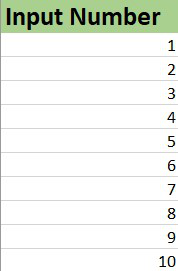### 1. Log base 10

To calculate the Antilog with base-10 we have to use the formula :

`10^Cell_number`

First, we calculate the log value of the given data using the Excel Logarithmic formula as discussed. Here, the numbers are stored in column number A, starting from A2.

The steps are :

1. Select the cell number where you want to find the log value.

2. Use the Excel formula to calculate log.

3. Click Enter.

4. The logarithmic will be displayed.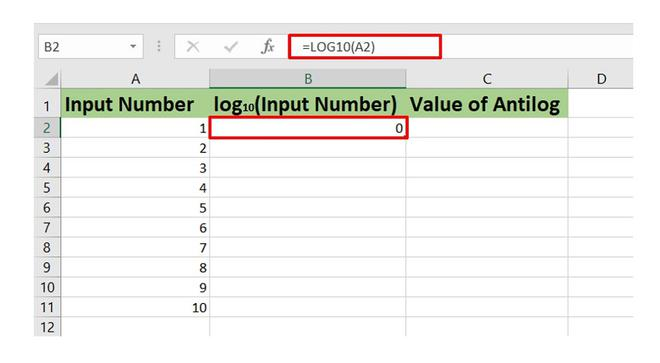Logarithmic Calculation of first ten natural numbers

Now, drag the Auto Fill Options to get the logarithmic value of the entire dataset in column B of the worksheet.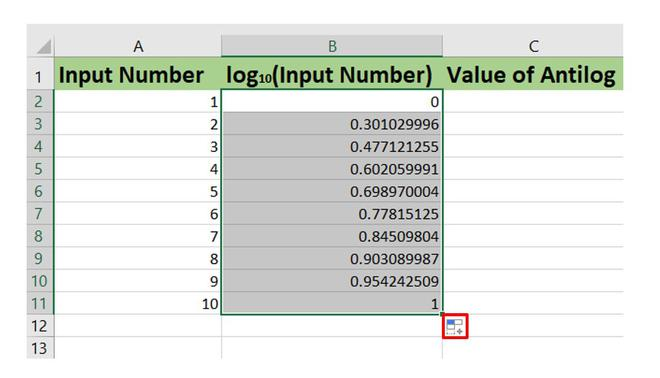Now, to calculate the antilog we need to write the antilog formula using the Exponent operator as shown below :

The steps are :

1. Select the cell number where you want to find the antilog value.

2. Use the Excel formula to calculate antilog.

3. Click Enter.

4. The antilog value will be displayed.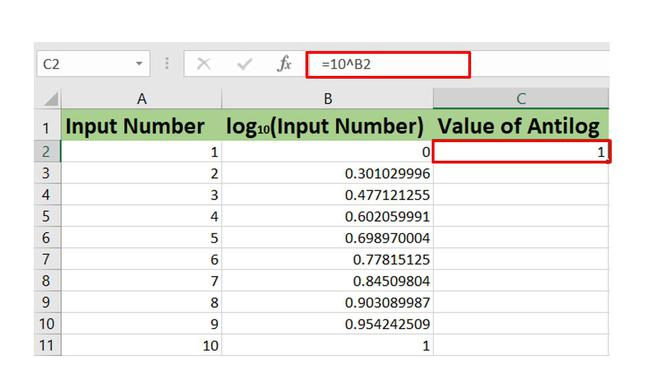Antilog

Again drag the Auto Fill Option to get the Antilog values.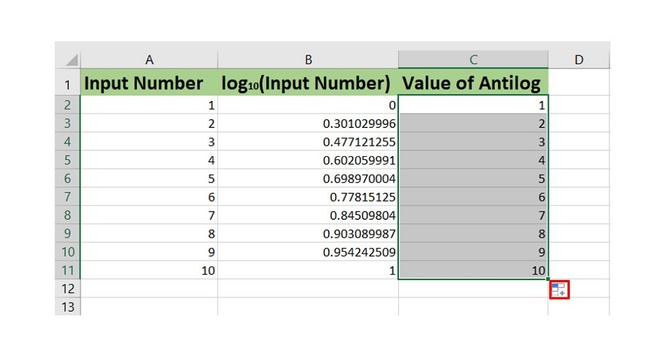Antilog values

### 2. Antilog of any random base

Say, now we need to calculate the log and antilog of the first ten natural numbers with base-2.

We will use the same steps as discussed in the previous section. Here, we have to use the Excel Formula :

`=LOG(Cell_Number,2)`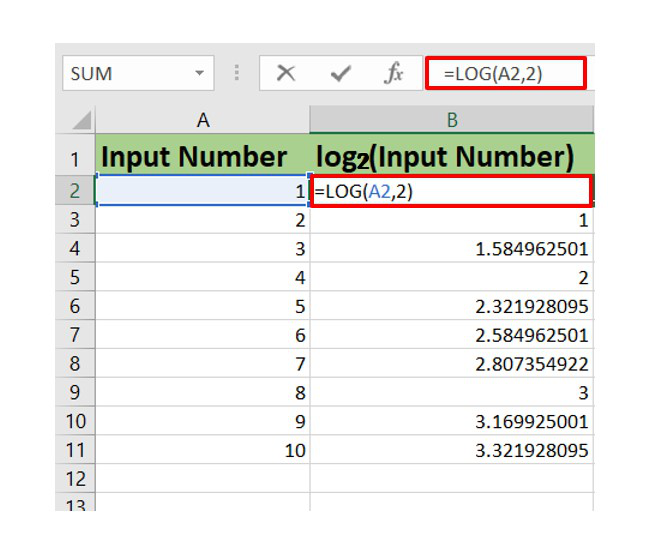Logarithm calculation

To calculate the Antilog use the formula :

`2^Cell_Number`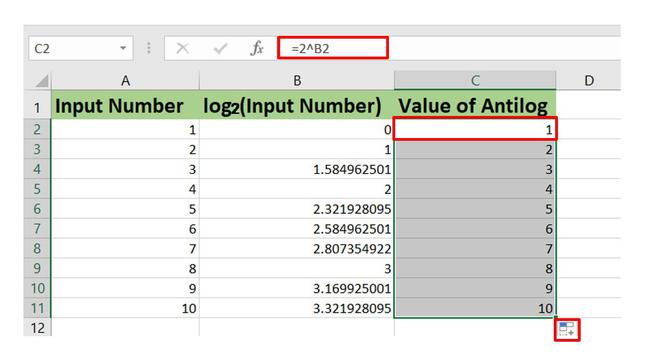### 3. Antilog of Natural logarithm values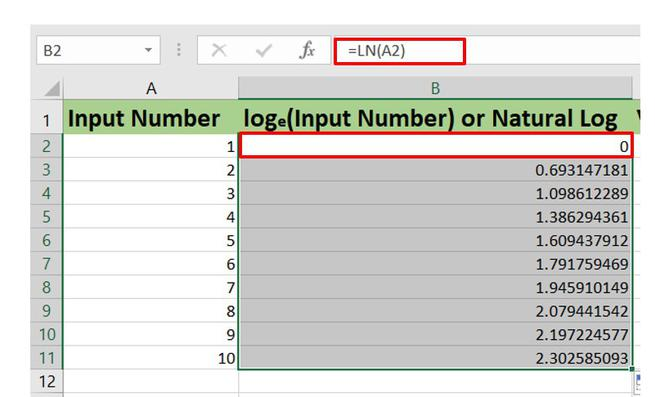Logarithm calculation

In Excel, the function used to calculate the power of the constant e is EXP. So, we need the EXP function in order to calculate the antilog of natural logarithmic values. The EXP function is basically the inverse of the LN function in Excel.

The syntax is :

`=EXP(Cell_number)`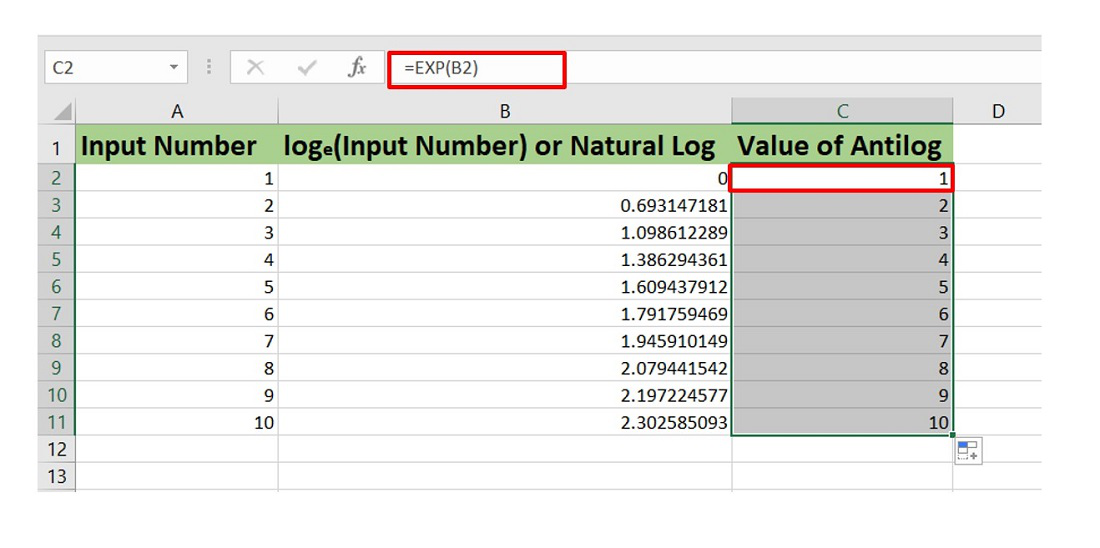My Personal Notes arrow_drop_up